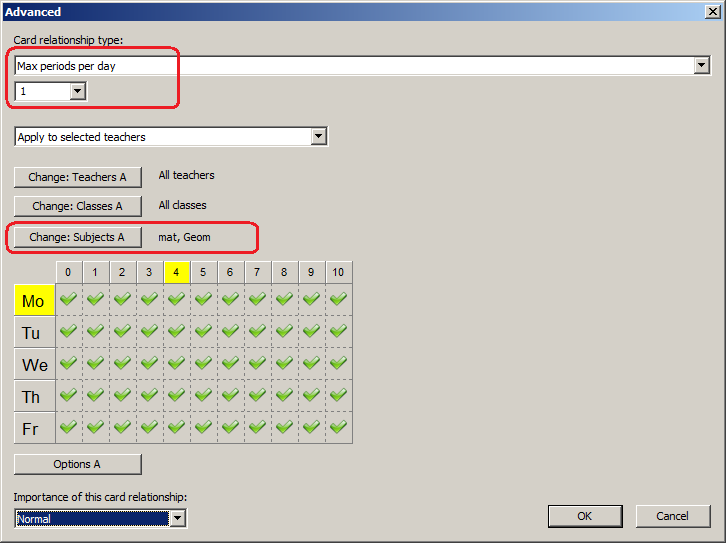aSc TimeTables - הגבלות - אחר

What does "Apply to" mean in the cardrelationships?
מאמר זה זמין בשפה :

When you are defining advanced cardrelationships you can specify the Apply to commbobox.

Lets suppose you specify condition max 1 Math or Geom per day:Now lets see what each apply to option will mean:

Apply Globally
There can be max 1 Math or Geom lesson per day in whole school. If Teacher Einstein have Math on Monday, no other teacher can have Math or Geom on Monday.

Apply to Teachers
Each teacher can have max 1 period of Math or Geom on each day. So if Einstein has Math on Monday, he cannot have Geom or another Math on Monday. But other teachers can have, of course, just one per teacher.
If you specify some teachers in the filter, only these teachers are considered. The other teachers are not affected at all.

Apply to Classes
Similiar to teachers, each class can have 1 Math or Geom per day.

Apply to Subjects in Selected classes
Each class can have max 1 math per day and max 1 Geom per day. So if 5A has Math, it cannot have another Math on the same day, but they can have 1 Geom.

Apply to classrooms
Each classroom will get max 1 math or geom per day.

Apply to students
Each student will get max 1 math or geom per day. So the class can have 5 math lessons per day each one for different students. This condition only works if you input students and courses. It doesn't work on regular groups.